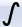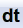Accessible through the “Signal tools/Integral curve” menu option (toolbar button) and “Signal tools/Differentiate” (toolbar button).

Integral curve

This option enables you to see how the integral of the signal changes through time. The result will be a new signal curve where the n-th value in this curve will represent the integral of the origin, signal from its beginning until its n-th sample.

If you need to only calculate a simple numerical integral of the signal (area under the signal curve), please use the corresponding option from the “Instruments and markers” menu.

Differentiate

This option calculates the first derivation of the signal by subtracting consecutive signal values from the origin values and forming a new curve from the subtraction results. This means that the n-th value in the new curve will be equal to the difference between the n-th and (n-1)-th value from the origin signal. This is only a rough approximation of the first derivation and should not be used on fast changing signals. Before applying this function to such a signal, you may try smoothing it first by using the appropriate Smoothingoption.